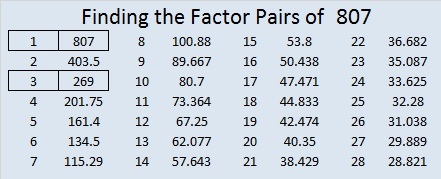# 1584 You Will L♥ve This Challenge Puzzle!

### Today’s Puzzle:

Here’s a Valentine’s challenge for you: Can you write the numbers from 1 to 10 in each of the four areas of the puzzle that touch the box with the X so that the numbers you write make the puzzle become four multiplication tables? It won’t be easy, but remember to use logic. If you succeed, you will absolutely lve the puzzle!### 1584 Factor Cake:

1584 obviously is divisible by 2. It is divisible by 4 because 84 is divisible by 4.
It is divisible by 8 because 84 is not divisible by 8 and 5 is odd.
1584 is divisible by 3 and by 9 because 1 + 5 + 8 + 4 = 18, a number divisible by 3 and by 9.
1584 is divisible by 11 because 1 – 5 + 8 – 4 = 0.

Thus, 1584 makes a very tall and very nice factor cake with a couple of candles on top!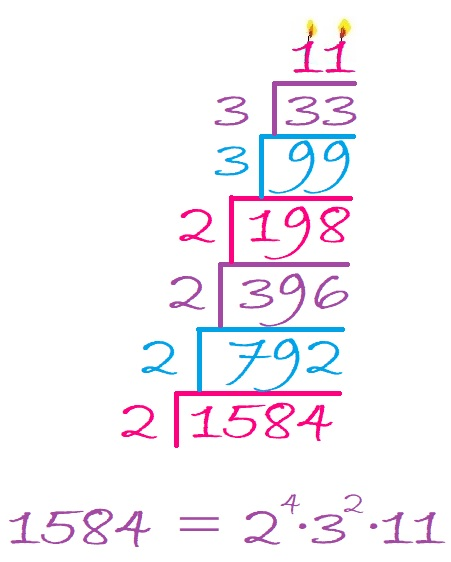### Factors of 1584:

• 1584 is a composite number.
• Prime factorization: 1584 = 2 × 2 × 2 × 2 × 3 × 3 × 11, which can be written 1584 = 2⁴ × 3² × 11.
• 1584 has at least one exponent greater than 1 in its prime factorization so √1584 can be simplified. Taking the factor pair from the factor pair table below with the largest square number factor, we get √1584 = (√144)(√11) = 12√11.
• The exponents in the prime factorization are 4, 2 and 1. Adding one to each exponent and multiplying we get (4 + 1)(2 + 1)(1 + 1) = 5 × 3 × 2 = 30. Therefore 1584 has exactly 30 factors.
• The factors of 1584 are outlined with their factor pair partners in the graphic below.### More about the Number 1584:

1584 is the difference of two squares NINE different ways:
397² – 395² = 1584,
200² – 196² = 1584,
135² – 129² = 1584,
103² – 95² = 1584,
72² – 60² = 1584,
53² – 35² = 1584,
47² – 25² = 1584,
45² – 21² = 1584, and
40² – 4² = 1584.
Yes, we are only 16 numbers away from 1600, the next perfect square!

1584 is in this cool pattern: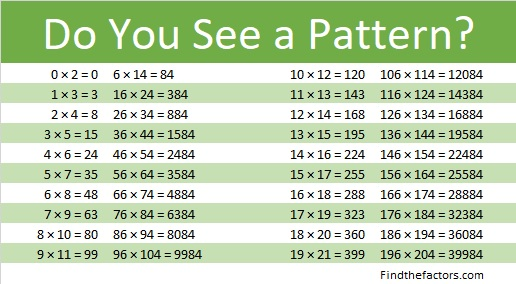# 1505 and Level 4

### Today’s Puzzle:

If you’ve never done a level 4 puzzle before, this one is a great choice. It has fewer tricky clues than usual. Start with a row or column that has two clues. Use logic and multiplication facts to figure out where to put the factors 1 to 10 in both the first column and the top row so that the given clues are the products of those factors. Have fun!### Factors of 1505:

• 1505 is a composite number.
• Prime factorization: 1505 = 5 × 7 × 43.
• 1505 has no exponents greater than 1 in its prime factorization, so √1505 cannot be simplified.
• The exponents in the prime factorization are 1, 1, and 1. Adding one to each exponent and multiplying we get (1 + 1)(1 + 1)(1 + 1) = 2 × 2 × 2 = 8. Therefore 1505 has exactly 8 factors.
• The factors of 1505 are outlined with their factor pair partners in the graphic below.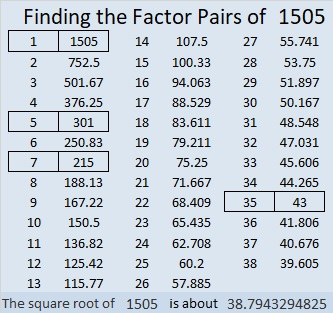### More about the Number 1505:

1505 has four different factor pairs. The numbers in each factor pair add up to an even number, so 1505 is the difference of two squares in four different ways. Here’s the complete list of those ways:
753² – 752² = 1505
153² – 148² = 1505
111² – 104² = 1505
39² – 4² = 1505

# 1504 and Level 3

### Today’s Puzzle:

Since this is a level 3 puzzle the clues are given in a logical order from top to bottom. Write the factors 1 to 10 in the first column and again in the top row.

Usually, you only have to consider the previous clues when finding the factors in a level 3 puzzle, but when you consider if 4 = 2 × 2 or 1 × 4, you will also have to look at a clue below it. You can do this!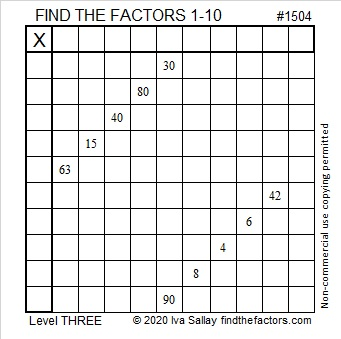### Factors of 1504:

• 1504 is a composite number.
• Prime factorization: 1504 = 2 × 2 × 2 × 2 × 2 × 47, which can be written 1504 = 2⁵ × 47.
• 1504 has at least one exponent greater than 1 in its prime factorization so √1504 can be simplified. Taking the factor pair from the factor pair table below with the largest square number factor, we get √1504 = (√16)(√94) = 4√94.
• The exponents in the prime factorization are 5 and 1. Adding one to each exponent and multiplying we get (5 + 1)(1 + 1) = 6 × 2 = 12. Therefore 1504 has exactly 12 factors.
• The factors of 1504 are outlined with their factor pair partners in the graphic below.### More about the Number 1504:

1504 is the difference of two squares in four different ways:
377² – 375² = 1504
190² – 186² = 1504
98² – 90² = 1504
55² – 39² = 1504

# 1468 and Level 1

### Today’s Puzzle

Can you find the factors from 1 to 12 that will work with the given clues to turn this puzzle into a multiplication table?### Factors of 1468

• 1468 is a composite number.
• Prime factorization: 1468 = 2 × 2 × 367, which can be written 1468 = 2² × 367
• 1468 has at least one exponent greater than 1 in its prime factorization so √1468 can be simplified. Taking the factor pair from the factor pair table below with the largest square number factor, we get √1468 = (√4)(√367) = 2√367
• The exponents in the prime factorization are 2 and 1. Adding one to each exponent and multiplying we get (2 + 1)(1 + 1) = 3 × 2 = 6. Therefore 1468 has exactly 6 factors.
• The factors of 1468 are outlined with their factor pair partners in the graphic below.### Another Interesting Fact about the number 1468:

OEIS.org informs us that the smallest 20-digit 6th power is 1468⁶.

# 1386 What You Need to Know About the Multiplication Game

### Helpful Hints about the Multiplication Game:

I recently wrote about Hooda Math’s Multiplication Game. There’s a couple of things I didn’t tell you in that post.

First of all, you don’t have to use a computer to play the game. (However, using one the first time you play will help you understand how to play). You can print a game board to play. I’ve created a game board below that you could use. Each player can use different items such as beads, pennies, nickels, and dimes as markers to mark the factors used and to claim the resulting products on the game board.The second thing you should know is that getting four squares in a row, horizontally, vertically or diagonally is NOT equally likely every place on the board. If one particular number is all you need to get a win, you are less likely to get that number if it only has one factor (like the numbers marked in yellow have). As far as this game is concerned, the products have the number of factors that I’ve indicated, even though in reality most of them have more than that.

You can’t win unless your opponent gives you one of the factors you need to claim that winning space. If 4 of the 9 possible factors will get it for you, the odds are much better your opponent will give you what you need than if only 1 of the 9 possible factors will do it.

If you know which numbers have four possible factors, you may have an advantage over someone who thinks this game is really just a variation of tic-tac-toe. Of course, those products with four factors could also make you more likely to get blocked as well! And if you use my colorful game board, your opponent will know just as much as you do about how many ways they can get each square.

### 1386 Factor Cake:

Since the biggest prime factor of 1386 is 11, it makes an especially festive factor cake!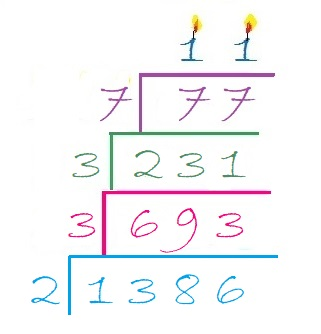### Factors of 1386:

Now I’ll share some information about the number 1386:

• 1386 is a composite number.
• Prime factorization: 1386 = 2 × 3 × 3 × 7 × 11, which can be written 1386 = 2 × 3² × 7 × 11
• 1386 has at least one exponent greater than 1 in its prime factorization so √1386 can be simplified. Taking the factor pair from the factor pair table below with the largest square number factor, we get √1386 = (√9)(√154) = 3√154
• The exponents in the prime factorization are 1, 2, 1, and 1. Adding one to each exponent and multiplying we get (1 + 1)(2 + 1)(1 + 1)(1 + 1) = 2 × 3 × 2 × 2 = 24. Therefore 1386 has exactly 24 factors.
• The factors of 1386 are outlined with their factor pair partners in the graphic below.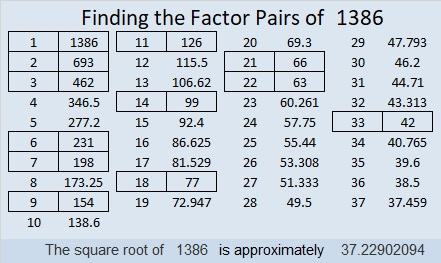### Sum-Difference Puzzle:

1386 has twelve factor pairs. One of the factor pairs adds up to 85, and a different one subtracts to 85. If you can identify those factor pairs, then you can solve this puzzle!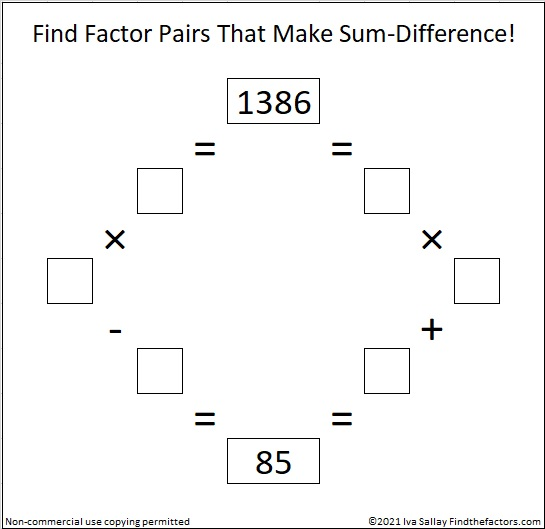### One More Fact about the Number 1386:

OEIS.org also noted that 1 + 3⁴ + 8 + 6⁴ = 1386.

# 1381 Hooda Math’s Multiplication Game

The school year is almost over, and class periods were only twenty-five minutes long today. I went online looking for math games that would benefit my students and I found a winner with Hooda Math’s Multiplication Game.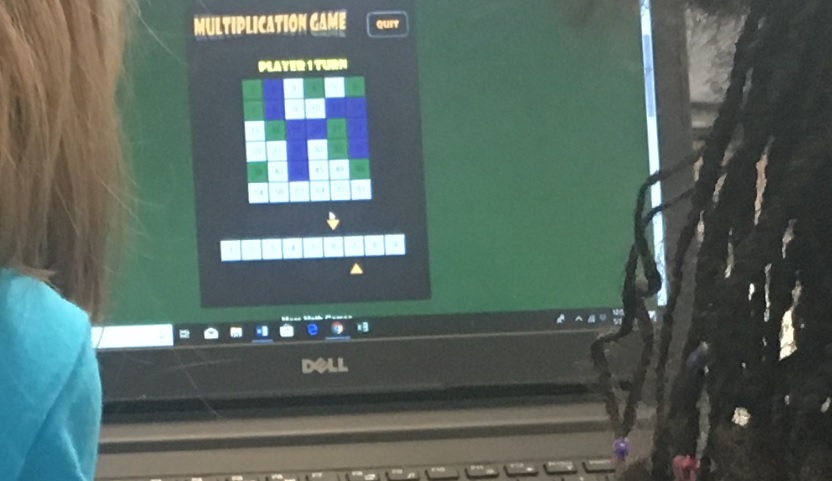If you count the multiplication facts in a 9×9 multiplication table, you will see 99 facts, but many of the products are duplicated in the table. Every yellow square below is also in white elsewhere in the table: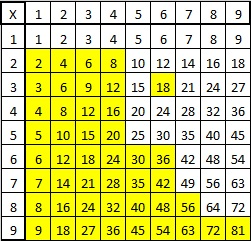There are actually only 36 unique products in the multiplication table above. Hooda Math has cleverly arranged those 36 products in a 6×6 grid that becomes the game board. In this two-person game, students take turns moving one of two arrows to a number from 1 to 9 at the bottom of the screen and claiming the square that contains the product of the numbers. The catch is that players must keep one of the numbers chosen by the previous player and cannot claim a product that has already been claimed by either player. (Player 1 cannot score on his first turn.) One student is green and the other is purple and the first to claim four squares in a row is the winner. The rules on the website are VERY short and simple.

Students played this game today. I played it as well. Sometimes I won, and sometimes I lost, but the losses are more interesting than the wins:

In one game, my opponent took the square that I needed to get four in a row vertically for the win. All she was trying to do was block me from winning, however, when she took that square, the game declared her the winner. We were puzzled why she was the winner until she figured out that making that move gave her four in a row diagonally. That’s when we found out players can win by getting four in a row diagonally as well as vertically or horizontally.

In another game, I had two possible moves that would have made me be the winner. I just needed my opponent to choose a 1, 6, or 8 as their other factor, and I would win with 1 × 1 = 1 or 6 × 8 = 48. Unfortunately, he knew to beware of the numbers that would make me win. One of the arrows was pointing to 5, and he made the other arrow point to 5. By now there were no other products left on the board that were divisible by 5, so I couldn’t win because I couldn’t move either of the arrows.

That’s how I didn’t win the game either of those times, but I had a lot of fun anyway, and you will, too!

This is my 1381st post. Here’s some information about that number:

• 1381 is a prime number.
• Prime factorization: 1381 is prime.
• 1381 has no exponents greater than 1 in its prime factorization, so √1381 cannot be simplified.
• The exponent in the prime factorization is 1. Adding one to that exponent we get (1 + 1) = 2. Therefore 1381 has exactly 2 factors.
• The factors of 1381 are outlined with their factor pair partners in the graphic below.How do we know that 1381 is a prime number? If 1381 were not a prime number, then it would be divisible by at least one prime number less than or equal to √1381. Since 1381 cannot be divided evenly by 2, 3, 5, 7, 11, 13, 17, 19, 23, 29, 31, or 37, we know that 1381 is a prime number.

1381 is the 24th Centered Pentagonal Number because
5(23)(24)/2 + 1 = 1381. In formula means that it is one more than five times the 23rd triangular number, as I’ve illustrated below:1381 is the sum of two squares:
34² +15² = 1381

1381 is the hypotenuse of a Pythagorean triple:
931-1020-1381 calculated from 34² -15², 2(34)(15), 34² +15²

Here’s another way we know that 1381 is a prime number: Since its last two digits divided by 4 leave a remainder of 1, and 34² + 15² = 1381 with 34 and 15 having no common prime factors, 1381 will be prime unless it is divisible by a prime number Pythagorean triple hypotenuse less than or equal to √1381. Since 1381 is not divisible by 5, 13, 17,  29, or 37, we know that 1381 is a prime number.

# 920 Witches’ Cauldron

“Double, double toil and trouble;
Fire burn, and caldron bubble.”

What besides “eye of newt” goes in witches’ cauldrons? The list includes some horrifying ingredients that you can read here from one scene from Shakeaspeare’s play, MacBeth.

Instead of putting “Eye of newt, and toe of frog, Wool of bat, and tongue of dog” and so forth in today’s Halloween cauldron puzzle, I just put a bunch of asterisks.Print the puzzles or type the solution on this excel file: 10-factors-914-922

“Double, double toil and trouble;
Fire burn, and caldron bubble.”

Double 115 is 230.

Double 230 is 460.

Double 460 is 920, today’s post number.

920 is the hypotenuse of a Pythagorean triple:
552-736-920 which is (3-4-5) times 184.

920 is palindrome 767 in BASE 11 because 7(121) + 6(11) + 7(1) = 920

• 920 is a composite number.
• Prime factorization: 920 = 2 × 2 × 2 × 5 × 23, which can be written 920 = 2³ × 5 × 23
• The exponents in the prime factorization are 3, 1, and 1. Adding one to each and multiplying we get (3 + 1)(1 + 1)(1 + 1) = 4 × 2 × 2 = 16. Therefore 920 has exactly 16 factors.
• Factors of 920: 1, 2, 4, 5, 8, 10, 20, 23, 40, 46, 92, 115, 184, 230, 460, 920
• Factor pairs: 920 = 1 × 920, 2 × 460, 4 × 230, 5 × 184, 8 × 115, 10 × 92, 20 × 46, or 23 × 40
• Taking the factor pair with the largest square number factor, we get √920 = (√4)(√230) = 2√230 ≈ 20.331501776.# 856 Rays of Light

When we learn something new, it is as if rays of light touch our minds. If you know how to multiply and divide, let this puzzle enlighten your mind. Just start at the top of the first column and work down cell by cell until the numbers 1 to 10 have been written in the first column and the top row and those corresponding numbers multiply together to give the clues in the puzzle.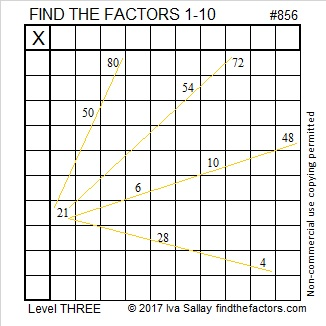Print the puzzles or type the solution on this excel file: 10-factors-853-863

• 856 is a composite number.
• Prime factorization: 856 = 2 × 2 × 2 × 107, which can be written 856 = 2³ × 107
• The exponents in the prime factorization are 3 and 1. Adding one to each and multiplying we get (3 + 1)(1 + 1) = 4 × 2 = 8. Therefore 856 has exactly 8 factors.
• Factors of 856: 1, 2, 4, 8, 107, 214, 428, 856
• Factor pairs: 856 = 1 × 856, 2 × 428, 4 × 214, or 8 × 107
• Taking the factor pair with the largest square number factor, we get √856 = (√4)(√214) = 2√214 ≈ 29.2574777Here are a few more advanced facts about the number 856:

856 is the 16th nonagonal number because 16(7⋅16-5)/2.

856 is the 19th centered pentagonal number because (5⋅19² + 5⋅19 + 2)/2 = 856.

OEIS.org informs us that if the Fibonacci sequence didn’t start with 1, 1, but instead started with 1, 9, we would get 1, 9, 10, 19, 29, 48, 77, 125, 202, 327, 529, 856, …

# 855 A Bottle Full of Multiplication Facts

If you’ve always wanted to know the multiplication facts better, there is hope for you to do that in this bottle! Just write the numbers from 1 to 10 in the top row and also in the first column in an order that makes those factors and the given clues act like a multiplication table.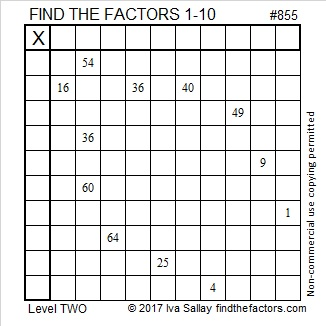Print the puzzles or type the solution on this excel file: 10-factors-853-863

855 is the hypotenuse of Pythagorean triple 513-684-855 which is (3, 4, 5) times 171.

From OEIS.org I learned that 855 can be expressed as sum of five consecutive squares (11² + 12² + 13² + 14² + 15² = 855) and the sum of two consecutive cubes (7³ + 8³ = 855). 855 is the smallest number that can make such a claim.

• 855 is a composite number.
• Prime factorization: 855 = 3 × 3 × 5 × 19, which can be written 855 = 3² × 5 × 19
• The exponents in the prime factorization are 2, 1, and 1. Adding one to each and multiplying we get (2 + 1)(1 + 1)(1 + 1) = 3 × 2 × 2 = 12. Therefore 855 has exactly 12 factors.
• Factors of 855: 1, 3, 5, 9, 15, 19, 45, 57, 95, 171, 285, 855
• Factor pairs: 855 = 1 × 855, 3 × 285, 5 × 171, 9 × 95, 15 × 57, or 19 × 45,
• Taking the factor pair with the largest square number factor, we get √855 = (√9)(√95) = 3√95 ≈ 29.240383# 807 and Level 1

What can I say about the number 807?

807 is palindrome 151 in BASE 26 because 1(26²) + 5(26) + 1(1) = 807.

Anything else? Well, I can figure out a few other things because 807’s has two prime factors, 3 and 269:

We can write ANY number (unless it’s a power of 2) as the sum of consecutive numbers in at least one way. 807 has three different ways to do that:

• 403 + 404 = 807 because 807 isn’t divisible by 2.
• 268 + 269 + 270 = 807 because it is divisible by 3.
• 132 + 133 + 134 + 135 + 136 + 137 = 807 since it is divisible by 3 but not by 6.

I know that one of 807’s factors, 269, is a hypotenuse of a Pythagorean triple, so 807 is also. Thus. . .

• (3·69)² + (3·260)² = (3·269)², or in other words, 207² + 780² = 807²

Since 807 has two odd sets of factor pairs, I know that 807 can be written as the difference of two squares two different ways:

• 136² – 133² = 807
• 404² – 403² = 807

I don’t usually do this, but today’s puzzle has something in common with 807. Can you tell what it is?Print the puzzles or type the solution on this excel file: 10-factors 807-814

• 807 is a composite number.
• Prime factorization: 807 = 3 x 269
• The exponents in the prime factorization are 1 and 1. Adding one to each and multiplying we get (1 + 1)(1 + 1) = 2 x 2 = 4. Therefore 807 has exactly 4 factors.
• Factors of 807: 1, 3, 269, 807
• Factor pairs: 807 = 1 x 807 or 3 x 269
• 807 has no square factors that allow its square root to be simplified. √807 ≈ 28.4077454